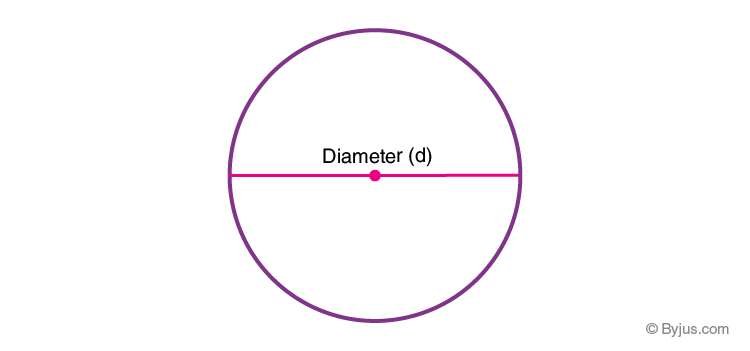# Diameter

In Geometry, we have studied two different types of shapes. They are 2D shapes and 3D shapes. Two-dimensional shapes have only two dimensions, such as length and breadth, whereas 3D shapes have three dimensions. Along with length and breadth, 3D shapes have height. The shapes are defined using different terms length, height, slant height, surface area, etc. One such parameter that defines the shape circle is “Diameter”. In this article, we are going to discuss the definition of diameter, diameter formulas with many solved examples.

## Diameter of a Circle

In Geometry, a circle is a 2D shape, where the collection of points on the surface of the circle is equidistant from the centre point. The distance from the centre to any point on the surface is called the radius. Similarly, the distance from one point on the surface of a circle to the other point on the surface of the circle through the centre is called the diameter. In other words, the diameter is double the radius. We can say that the diameter is the longest chord of a circle. The notations used to represent diameter are ‘d’, ‘φ’, ‘D’, ‘Dia’.## Diameter Formula

The different ways to calculate the diameter of a circle are given below:

If the radius of a circle is known, the diameter is calculated as:

D= 2R

Where “R” is the radius of the circle

If the circumference of a circle is known, then the formula to calculate the diameter is

D = C/π

Where,

C is the circumference of a circle

π is a constant value, which is approximately equal to 3.14.

If the area of a circle is known, then the formula to compute the diameter of a circle is

$$\begin{array}{l}D = \sqrt{\frac{4A}{\pi }}\end{array}$$

(or)

$$\begin{array}{l}D = 2\sqrt{\frac{A}{\pi }}\end{array}$$

Where,

A is the area of a circle

## Diameter Properties

The properties of the diameter of a circle are as follows:

• The diameter is the longest chord of any circle.
• The diameter divides the circle into two equal parts and thus produces two equal semicircles.
• The midpoint of the diameter is the centre of the circle.
• The radius should be half of the diameter.

### Solved Examples

Go through the following problems to find the diameter of a circle.

Example 1:

Find the diameter of a circle if its radius is 3 cm.

Solution:

Given:

We know that, if the radius is given, the formula to calculate the diameter is:

D = 2R.

Substitute R = 3 cm in the formula, we get

D = 2(3)

D = 6 cm.

Example 2:

The circumference of a circle is 36 cm. Calculate the diameter of a circle.

Solution:

Given:

Circumference, C = 36 cm.

We know that,

D = C/π

Now, substitute C = 36 cm and π= 3.14 in the formula,

D = 36/3.14

D = 11.5 (approximately)

Hence, the diameter of a circle is approximately equal to 11.5 cm.

Example 3:

Determine the diameter of a circle, given that the area is 125 cm2.

Solution:

Given:

Area of a circle, A = 125 cm2.

If the circle’s area is given, then the formula to calculate the diameter of a circle is given by:

$$\begin{array}{l}D = 2\sqrt{\frac{A}{\pi }}\end{array}$$

Now, substitute the value of A and π in the formula, we get

$$\begin{array}{l}D = 2\sqrt{\frac{125}{3.14}}\end{array}$$

D= 2(6.31)

D = 12.62 (approximately)

Thus, the diameter of a circle is 12.62 cm.

### Practice Problems

Calculate the diameter of the circle, if:

2. Area = 169 cm2.
3. Circumference = 144 cm.

## Frequently Asked Questions on Diameter

Q1

### What is the diameter of a circle?

The diameter of a circle is the distance from one point on the surface of a circle to the other point on the circle’s surface, which passes through the centre.

Q2

### Is the diameter the longest chord of a circle?

Yes, the diameter is the longest chord of a circle.

Q3

### Calculate the radius of a circle if its diameter is 4 cm.

If the diameter is 4 cm, then the radius is 2 cm. (i.e.) R= D/2.

Q4

### What is the formula to calculate the diameter of the circumference of a circle is given?

If the circumference of a circle is given, then the formula to compute the diameter is:

Diameter = Circumference/π

Q5

### Is the diameter double the radius of a circle?

Yes, the diameter of a circle is double its radius. If D is the diameter, R is the radius, then D= 2R.